Next: Estimating the Fundamental Matrix Up: Epipolar Geometry Previous: Basic Considerations

## The Fundamental Matrix

Let m = (x, y, t)t be the homogeneous coordinates of a point in the first image and e = (u, v, w) be the coordinates of the epipole of the second camera in the first image. The epipolar line through m and e is represented by the vector(c.f. section 2.2.1). The mapping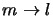is linear and can be represented by a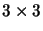rank 2 matrix C: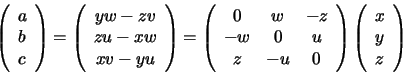(5.1)

The mapping of epipolar lines l from image 1 to the corresponding epipolar lines l' in image 2 is a collineation defined on the 1D pencil of lines through e in image 1. It can be represented (non-uniquely) as a collineation on the entire dual space of lines in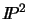. Let A be one such collineation: l'= A l.

The constraints on A are encapsulated by the correspondence of 3 distinct epipolar lines. The first two correspondences each provide two constraints, because a line in the plane has 2 dof. The third line must pass through the intersection of the first two, so only provides one further constraint. The correspondence of any further epipolar line is then determined, for example by its cross ratio with the three initial lines. Since A has eight degrees of freedom and we only have five constraints, it is not fully determined. Nevertheless, the matrix F = A C is fully determined. Using (5.1) we get

 l' = ACm=Fm (5.2)

F is called the fundamental matrix. As C has rank 2 and Ahas rank 3, F has rank 2. The right kernel of C -- and hence F-- is obviously the epipole e. The fact that all epipolar lines in the second image pass through e' (i.e.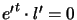for all transferred l') shows that the left kernel of F is e':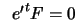.

F defines a bilinear constraint between the coordinates of corresponding image points. If m' is the point in the second image corresponding to m, it must lie on the epipolar line l'=Fm, and hence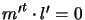(c.f. 5.1). The epipolar constraint can therefore be written:

 m't F m=0 (5.3)Next: Estimating the Fundamental Matrix Up: Epipolar Geometry Previous: Basic Considerations
Bill Triggs
1998-11-13